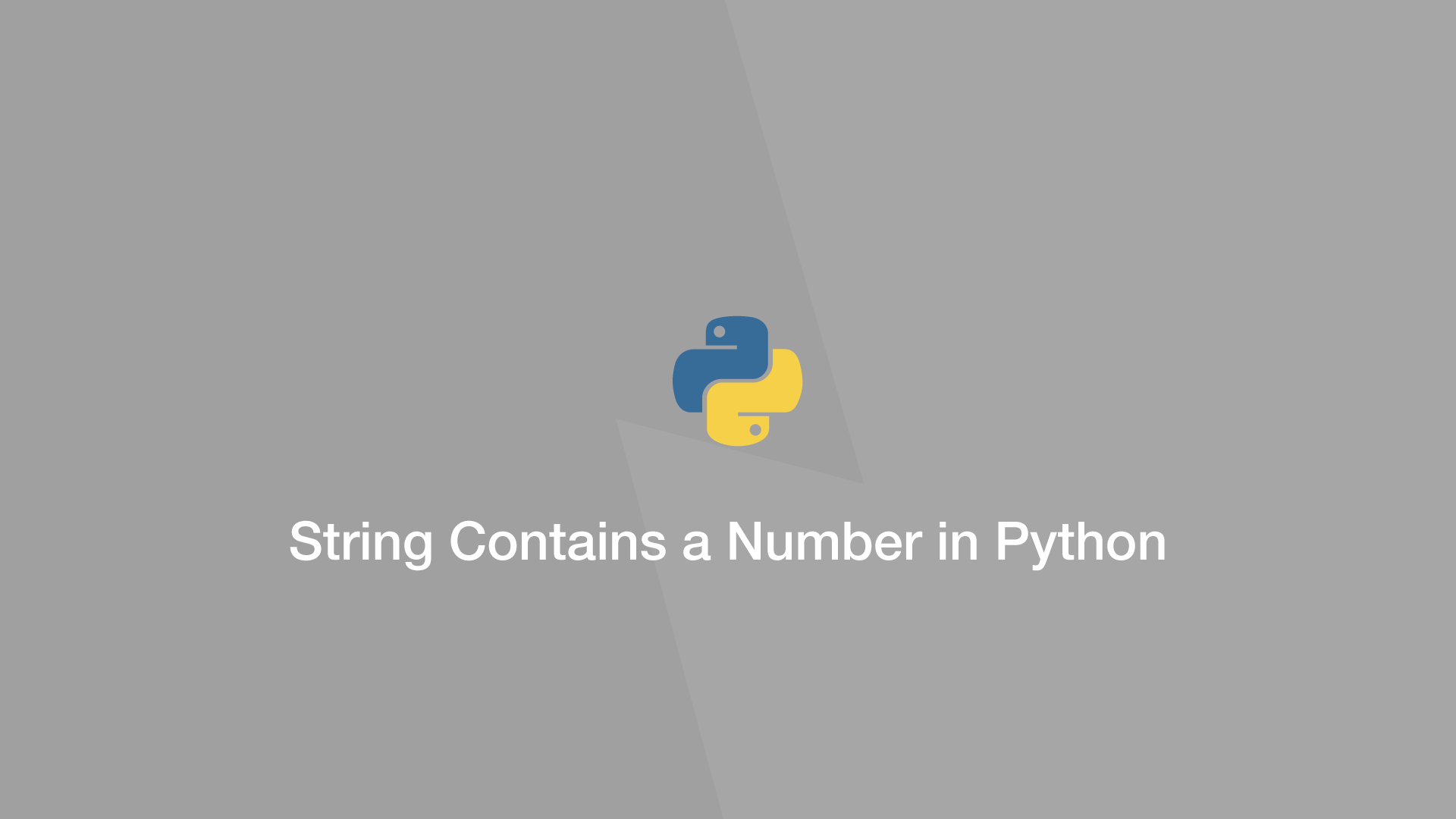# How to Check if a String Contains a Number in PythonIn this tutorial, we will learn how to check if a string contains a number in Python.

## Check Each Character with str.isdigit()

The first way we can approach solving this problem is to loop through and evaluate each character with the `str.isdigit()` function. If the character is indeed a number `.isdigit()` will return True.

``````string = 'Some t3xt'
result = False

for i in string:
if str.isdigit(i):
result = True

print(result)
``````
``````True
``````

To do the above operation in one line, use the Python `any()` function which returns `True` if anything in an iterable returns `True`.

``````string = 'Some t3xt'

print(any(str.isdigit(i) for i in string))
``````
``````True
``````

## Check if String Contains a Number with map()

Instead of a for loop we can use the Python `map()` function, which will do the same job but with a cleaner syntax.

``````string = 'Some t3xt'

print(any(map(str.isdigit, string)))
``````
``````True
``````

If you prefer to use RegEx to evaluate strings, import the `re` package and use the `.search()` function. Pass an expression that will for a number in the string as the first argument and the string as the second like this:

``````import re

string = 'Some t3xt'

print(re.search(r'\d', string))
``````
``````<re.Match object; span=(6, 7), match='3'>
``````

If a RegEx match is found `.search()` will return a `re.Match` object, otherwise, `None` is returned.

## Get Position of a Number in a String and the Value of the Number

The advantage of using the above method is that the `re.Match` object contains data about the position of the number found in the string and what that number is.

``````import re

string = 'Some t3xt'

result = re.search(r'\d', string)

print(result.group(0))
print(result.span())
``````
``````3
(6,7)
``````

`group(0)` returns the matching value and `span()` returns its position in the string.

#### Related Tutorials### How to Convert a String to a Number in Python

October 01, 2020### How to Check if a String Contains a Substring in Python

September 21, 2020### How to use Sets in Python

September 15, 2020### How to Compare Strings in Python

September 20, 2020### How to Create and Use Arrays in JavaScript

July 11, 2020### How to use slice() & substring() to Get Parts of a String in JavaScript

September 12, 2020